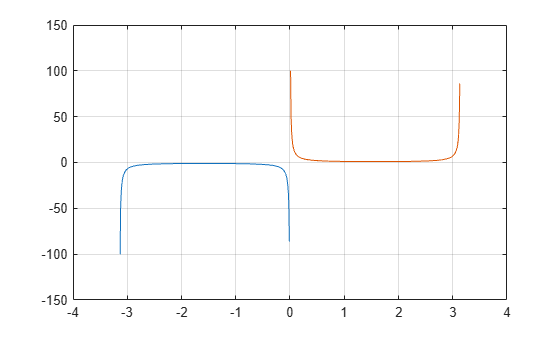# csc

Cosecant of input angle in radians

## Syntax

``Y = csc(X)``

## Description

example

````Y = csc(X)` returns the cosecant of the elements of `X`. The `csc` function operates element-wise on arrays. The function accepts both real and complex inputs. For real values of `X`, `csc(X)` returns real values in the interval [-∞, -1] and [1, ∞].For complex values of `X`, `csc(X)` returns complex values. ```

## Examples

collapse all

Plot the cosecant function over the domain $-\pi and $0 as shown.

```x1 = -pi+0.01:0.01:-0.01; x2 = 0.01:0.01:pi-0.01; plot(x1,csc(x1),x2,csc(x2)), grid on```Calculate the cosecant of the complex angles in vector `x`.

```x = [-i pi+i*pi/2 -1+i*4]; y = csc(x)```
```y = 1×3 complex 0.0000 + 0.8509i 0.0000 + 0.4345i -0.0308 - 0.0198i ```

## Input Arguments

collapse all

Input angle in radians, specified as a scalar, vector, matrix, or multidimensional array.

Data Types: `single` | `double`
Complex Number Support: Yes

## Output Arguments

collapse all

Cosecant of input angle, returned as a real-valued or complex-valued scalar, vector, matrix or multidimensional array.

collapse all

### Cosecant Function

The cosecant of an angle, α, defined with reference to a right angled triangle isThe cosecant of a complex argument, α, is

`$\text{csc}\left(\alpha \right)=\frac{2i}{{e}^{i\alpha }-{e}^{-i\alpha }}\text{\hspace{0.17em}}.$`

## Tips

• In floating-point arithmetic, `csc` is a bounded function. That is, `csc` does not return values of `Inf` or `-Inf` at points of divergence that are multiples of `pi`, but a large magnitude number instead. This stems from the inaccuracy of the floating-point representation of π.